# Nuts

How many we must have at least nuts if we can equally divide it to 10 children, 12 children or 15 children and any nut left?

n =  60

### Step-by-step explanation: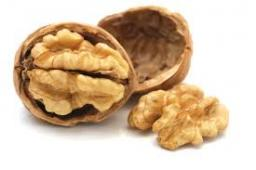Did you find an error or inaccuracy? Feel free to write us. Thank you!Tips to related online calculators
Do you want to calculate least common multiple two or more numbers?

## Related math problems and questions:

• PlumsIn the bowl are plums. How many would be there if we can divide it equally among 8, 10 and 11 children?
• CherriesCherries in the bowl can be divided equally among 3 or 15 or 5 children. How many are the minimum cherries in the bowl?
• Plum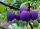On the platter are plum. How many were there if its have to be able to share equally among 8,10 and 12 children?
• School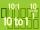Headteacher think whether the distribution of pupils in race in groups of 4,5,6,9 or 10. How many pupils must have at least school at possible options?
• Parachutists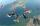Parachutists during freefall firstly held in groups of 4, then of 6, then 9, 12 and finally of 18 members. How many parachutists jump at least should be, if at each group must all be involved.
• TilesFrom how many tiles 20 cm by 30 cm, we can build a square of maximum dimensions if we have maximum 881 tiles.
• Four poplarsFour poplars are growing along the way. The distances between them are 35 m, 14 m, and 91 m. At least how many poplars need to be dropped to create the same spacing between the trees? How many meters will it be?
• LCMWhat is the least common multiple of 5, 50, 14?
• Apples and pearsMom divided 24 apples and 15 pears to children. Each child received the same number of apples and pears - same number as his siblings. How many apples (j=?) and pears (h=?) received each child?
• A ropeA rope can be cut into equal length with no rope left over. The lengths can be 15cm,18cm or 25cm. What is the shortest possible length of the rope?
• Lcm to eliminate yIdentify the least common multiple that would eliminate the y-variable. 6x - 5y = -4 4x + 2y = 28
• Lcm = 22 + gcdThe least common multiple of two numbers is 22 more than their greatest common divisor. Find these numbers.
• PexesoDrawing paper has dimensions of 60cm and 840mm. Pupils have to divide it into squares so that they can make a pexeso. What dimension must squares have if their side is to be larger than 3cm and less than 10cm?
• DivisibilityDetermine the smallest integer which divided 11 gives remainder 4 when divided 15 gives remainder 10 and when divided by 19 gives remainder 16.
• Lcm of three numbersWhat is the Lcm of 120 15 and 5
• Matches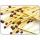George poured out of the box matches and composing them triangles and no match was left. Then he tries squares, hexagons and octagons and no match was left. How many matches must be at least in the box?
• Summer camp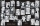Some boys or girls signed up for the summer camp, which has a maximum capacity of 200 children. The main leader noticed that during the evening start, he could arrange the participants exactly in the twelve-step, sixteen-step, or eighteen-step, and no one